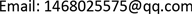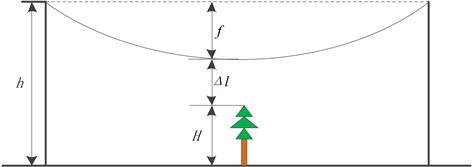﻿ 输电线路树闪故障分析及树线距离预测方法研究 Research on Tree Flash Failure Analysis and Tree Line Distance Prediction Method for Transmission Line

Journal of Electrical Engineering
Vol. 07  No. 02 ( 2019 ), Article ID: 30675 , 6 pages
10.12677/JEE.2019.72010

Research on Tree Flash Failure Analysis and Tree Line Distance Prediction Method for Transmission Line

He Liu

Electric Power Research Institute of State Grid Corporation of Jilin, Changchun JilinReceived: May 15th, 2019; accepted: May 30th, 2019; published: June 6th, 2019ABSTRACT

In order to cope with the tree flash failure caused by the ultra-high trees under the line, the tree line distance prediction factor is established by analyzing the hidden troubles of the tree flash fault. On this basis, the tree growth model is established according to tree type and site conditions, and the cable sag change model is established according to the stress change and temperature change of transmission line. The above two models are used to predict the spacing between tree lines and improve the safety of power grid operation.

Keywords:Tree Flash Failure, Tree Line Spacing, Tree Growth Model, Sag Variation Model1. 引言

2. 树闪故障分析

1) 在雨雪雷电等灾害天气情况下，输电走廊下方及周边的树木或断枝触碰电力线路，可能引发树闪故障甚至断线。对于灾害天气，电网有专门的应急机制，运维人员可以随时对故障情况做出反应并处理。

2) 在大风情况下，电力线路和树木都发生晃动，在晃动幅度较大使得树线安全间距不足时，线路就可能与电力走廊外的树木之间发生树闪故障。这种情况的树闪故障可能较多，但一般为瞬时性故障，自动重合闸一般能保证线路继续运行，不会造成重大的系统故障。

3) 在线路负荷较大的情况下，使得导线温度升高，从而使输电线路弧垂增加，尤其在夏季，不仅用电负荷会增大，也使导线散热减慢，线路弧垂增加幅度更大。这种情况下由树线安全间距不足引发树闪故障跳闸时，即使线路通过自动重合闸短时恢复运行也会由于导线过热下垂树闪而再度跳闸退出运行。当一条线路由于退出运行使得负荷转移到其它线路上时，容易使得其它线路过热而弧垂增加，造成连锁故障的发生。

3. 树线间距预测模型

3.1. 树木生长模型

$\text{d}H/\text{d}t=K{\left(1-{\text{e}}^{-Am}\right)}^{{A}_{1}}$ (1)

$H=K{\left[1-{\text{e}}^{-m\left(t-{t}_{0}\right)}\right]}^{n}$ (2)

3.2. 导线弧垂变化模型

${\sigma }_{t}^{3}+A{\sigma }_{t}^{2}+B=\text{0}$ (3-1)

$A=-\left[{\sigma }_{0}-\frac{E{\gamma }_{0}^{2}{l}^{2}}{24{\sigma }_{0}^{2}}-\alpha E\left({\int }_{{T}_{0}}^{T}\frac{R{I}^{2}}{m{C}_{p}}\text{d}t\right)\right]$ (3-2)

$B=\frac{E{\gamma }_{t}^{2}{L}^{2}}{24}$ (3-3)

$f=\frac{{L}^{2}{\gamma }_{t}}{8{\sigma }_{t}\mathrm{cos}\beta }$ (4)

3.3. 树线间距

$\Delta l=h-H-f$ (5)

4. 应用结果分析

4.1. 树木生长模型Figure 1. Tree line spacing calculation principle

$H=-a{x}^{2}+bx+c$ (6)

$\lambda =\frac{\mathrm{lg}{H}_{2}-\mathrm{lg}{H}_{1}}{\mathrm{lg}{H}_{3}-\mathrm{lg}{H}_{1}}$ (7)

$H=20.752{\left(1-{\text{e}}^{-0.239t}\right)}^{10.325}$ (8)

4.2. 导线弧垂计算

4.3. 树线距离预测

5. 结论

Research on Tree Flash Failure Analysis and Tree Line Distance Prediction Method for Transmission Line[J]. 电气工程, 2019, 07(02): 92-97. https://doi.org/10.12677/JEE.2019.72010

1. 1. 刘怀东, 陈伟, 高晓辉, 等. 从大停电观点分析一类输电线路树闪故障[J]. 电网技术, 2007(31): 67-69.

2. 2. 胡毅, 刘凯, 吴田, 等. 输电线路运行安全影响因素分析及防治措施[J]. 高电压技术, 2014, 40(11): 3491-3499.

3. 3. Wit, E. and McClure, J. (2004) Statistics for Microarrays: Design, Analysis, and Inference. 5th Edition, John Wiley & Sons Ltd., Chichester, 5-18.

4. 4. 甘德强, 胡江溢, 韩祯祥. 2003年国际若干停电事故思考[J]. 电力系统自动化, 2004, 8(3): 1-4, 9.

5. 5. 祝贺, 于卓鑫, 严俊韬. 特高压输电线路树障隐患预判及仿真分析[J]. 东北电力大学学报, 2018, 38(2): 21-27.

6. 6. 杨楠, 刘晓波. 基于树木生长模型的输电线路通道灾害预警方法的研究[J]. 新型工业化, 2017, 7(12): 11-15.

7. 7. 马林. 有效改善架空线路弧垂的策略及输送容量研究[D]: [硕士学位论文]. 天津: 天津大学, 2016.

8. 8. 中华人民共和国住房和城乡建设部, 中华人民共和国国家质量监督检验检疫总局. 110~750 kV架空输电线路设计规范: GB50545-2010 [S]. 北京: 中国计划出版社, 2010.